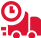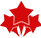# MAT240S: The following data was collected from a random sample of students These data represent their commuting distance (in km) per week: math’s statistics Assignment, SU, Malaysia

 University Sunway University (SU) Subject MAT240S: math statistics

Calculate the sample

a) mean, median, and mode.

b) variance and standard deviation.

c) coefficient of variation.

d) Pearson coefficient of skewness.

Based on the skewness value, comment on the shape of the distribution.

2. The following data were collected from a random sample of students. These data represent their commuting distance (in km) per week.

Calculate the sample

a) mean, median, and mode.

b) variance and standard deviation.

c) coefficient of skewness.

d) coefficient of variation.

e) quartiles (Q1, Q2, Q3), using the percentile formula.

f) Comparing the distribution of the datasets in Questions 1 and 2, which one is less dispersed? Justify your answer.

3. Identify the type of data (level of measurement), for each variable in the data set: (Ratio / Nominal or Ordinal)

4. For the dataset student_profile.xlsx, choose one (1) ratio variable.

a) Form an ungrouped frequency table, showing the frequency, cumulative frequency and the relative frequency.

b) Create a fully- labelled box-plot to summarize the data.

c) Are there any mild or extreme outliers for this ratio variable? If there are, what are the values? Justify your answer. (Use the outlier boundary formula)

d) Write a short summary to explain the graph. Include the shape of the distribution.

5. For the dataset student_profile.xlsx, choose another (1) ratio variable.

a) Form a grouped frequency table with 6 classes.

b) Describe the data in a fully-labeled histogram.

c) Write a short summary to explain the graph. Include the shape of the distribution.

6. For the dataset student_profile.xlsx, choose one (1) nominal variable that has at least 4 categories.

a) Form a frequency table, showing the frequency and the relative frequency (proportion).

b) Create a suitable graph (fully-labeled) to summarize the data. (4m) c) Write a short summary to explain the graph.

7. For the dataset student_profile.xlsx, choose another one (1) nominal variable and one (1) ordinal variable. Choose variables that have at least 3 categories.
Form a contingency table to show the relationship between the two variables.

8. For the dataset student_profile.xlsx, choose two (2) ratio variables.

a) Create a chart to display the relationship between the two variables.

b)  Use EXCEL function to calculate the Pearson correlation coefficient.

Based on the coefficient value, explain the relationship between the two variables. )

9. For the dataset student_profile.xlsx, choose another two (2) ratio variables (different from those used in Question 8).

a) Use EXCEL function to calculate the sample mean, median, mode, variance and standard deviation of each variable.

b) Use EXCEL function to calculate the Pearson coefficient of skewness of each variable.

c) Use the EXCEL function to calculate the coefficient of variation of each variable.

d) Comparing the distribution of the two variables, which one is less dispersed?

## Get Help By ExpertOnline Exam & Assignment Writing Services11770+ Orders Delivered4.9/5
5 Star Rating

Confidential & Secure Assignment Help For

Group Assignment Help

Online Exam -Test & Quiz

Cheapest Price Quote

Diploma & Certificate Levels

Semester & FYP Papers

Summative & Individual

GBA & Reflective

Last Minute Assistance

##### LAW299 Business Law Assignment Sample UiTM Malaysia
Business Law is a complicated subject matter that has been studied for hundreds, if not thousands of years. This course provides an overview of the major aspects including legal organization…
Law
##### MGT300 Information Technology In Business Assignment Example UiTM Malaysia
The world is changing with the advancement in technology and today's e-business enterprises must be able to adapt quickly. The course discusses how IT plays an important role when making…